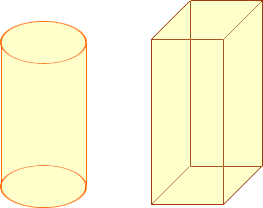Quandaries and Queries Hi, I try to find the measurement for a juice jug. I have the height of the jug and need the jug to hold 2.5 Liter of juice. Can you help me out with the formula. Thanks. school project Edward Hi Edward, The answer depends on the shape of the juice jug. I assume your jug has straight sides that are perpendicular to the base, but I don't know the shape of the base. It might have a circular base like the image on the left below, or a square base like the image on the right. I have a friend with a juice jug where the shape of the base is a triangle. These shapes are called cylinders, the one on the left is a circular cylinder and the one on the right is a square cylinder. My friend's jug is in the shape of a triangular cylinder.The volume of a cylinder is the area of the base times the height and hence if you can find the area of the base you can calculate the volume. One liter is 1,000 cubic centimeters so 2.5 liters is 2,500 cubic centimeters. Hence, if the height of the jug is h centimeters, the jug will have a volume of 2.5 liters if the area of the base is  2,500/h square centimeters. Cheers, Penny Go to Math Central